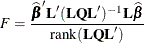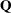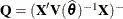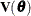#### Type III Tests of Fixed Effects

The Type III Tests of Fixed Effects table contains hypothesis tests for the significance of each of the fixed effects specified in the MODEL statement. By default, PROC GLIMMIX computes these tests by first constructing a Type IIImatrix for each effect; see Chapter 15: The Four Types of Estimable Functions. Thematrix is then used to construct the test statisticwhere the matrixdepends on the estimation method and options. For example, in a GLMM, the default is, whereis the marginal variance of the pseudo-response. If you specify the DDFM=KENWARDROGER option,is the estimated variance matrix of the fixed effects, adjusted by the method of Kenward and Roger (1997). If the EMPIRICAL= option is in effect,corresponds to the selected sandwich estimator.

You can use the HTYPE= option in the MODEL statement to obtain tables of Type I (sequential) tests and Type II (adjusted) tests in addition to or instead of the table of Type III (partial) tests.

The ODS names of the Type I Tests of Fixed Effects through the Type III Tests of Fixed Effects tables are Tests1 through Tests3, respectively.